Updating search results...

# 7 Results

View
Selected filters:
• UT.MATH.I.SI.MP.7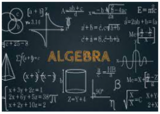Conditional Remix & Share Permitted
CC BY-NC
Rating
0.0 stars

This unit was created as an introductory/review unit for Algebra II to allow for a review of main concepts needed to progress in Algebra II. However, it could also be used in Algebra I to introduce for the first time real numbers;&nbsp;number operations;&nbsp;and equations, expressions, and inequalities.

Subject:
Secondary Mathematics
Material Type:
Homework/Assignment
Lesson
Unit of Study
Author:
Emily
02/22/2022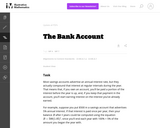Unrestricted Use
CC BY
Rating
0.0 stars

Subject:
Mathematics
Secondary Mathematics
Material Type:
Activity/Lab
Provider:
Illustrative Mathematics
Provider Set:
Illustrative Mathematics
Author:
Illustrative Mathematics
05/01/2012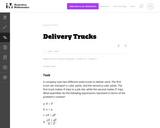Unrestricted Use
CC BY
Rating
0.0 stars

The primary purpose of this task is to illustrate certain aspects of the mathematics described in the A.SSE.1. The task has students look for structure in algebraic expressions related to a context, and asks them to relate that structure to the context. In particular, it is worth emphasizing that the task requires no algebraic manipulation from the students.

Subject:
Mathematics
Secondary Mathematics
Material Type:
Activity/Lab
Provider:
Illustrative Mathematics
Provider Set:
Illustrative Mathematics
Author:
Illustrative Mathematics
05/01/2012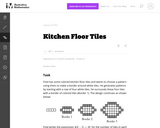Unrestricted Use
CC BY
Rating
0.0 stars

The purpose of this task is to give students practice in reading, analyzing, and constructing algebraic expressions, attending to the relationship between the form of an expression and the context from which it arises. The context here is intentionally thin; the point is not to provide a practical application to kitchen floors, but to give a framework that imbues the expressions with an external meaning.

Subject:
Mathematics
Secondary Mathematics
Material Type:
Activity/Lab
Provider:
Illustrative Mathematics
Provider Set:
Illustrative Mathematics
Author:
Illustrative Mathematics
05/01/2012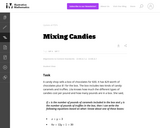Unrestricted Use
CC BY
Rating
0.0 stars

This task assumes students are familiar with mixing problems. This approach brings out different issues than simply asking students to solve a mixing problem, which they can often set up using patterns rather than thinking about the meaning of each part of the equations.

Subject:
Mathematics
Secondary Mathematics
Material Type:
Activity/Lab
Provider:
Illustrative Mathematics
Provider Set:
Illustrative Mathematics
Author:
Illustrative Mathematics
05/01/2012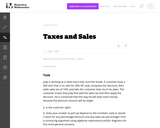Unrestricted Use
CC BY
Rating
0.0 stars

This task is not about computing the final price of the shirt but about using the structure in the computation to make a general argument.

Subject:
Mathematics
Secondary Mathematics
Material Type:
Activity/Lab
Provider:
Illustrative Mathematics
Provider Set:
Illustrative Mathematics
Author:
Illustrative Mathematics